www.batmath.it

### Continuity

Roughly speaking: a function is continuous if we can draw its graph without lifting our pencil from the paper.  This "definition" makes little sense from a mathematical point of view, so we investigate it with greater detail.

First of all the formal definition:Given a function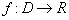, and a point c in the domain D, the function f is said to be continuous at c if either c is not an accumulation point for D, or, when c is an accumulation point,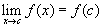.

Important remarks

The point c must be in the domain of the function.
The value l that we used in the definition of limit is now replaced by f(c).

Unlike the case of limits, here we do not want to investigate the behaviour of a function as x gets closer and closer to a given point c; on the contrary we want to check if the function approaches exactly the value f(c) as x approaches c.

Observe that, if c is a left (right) end point in the domain, we need to check only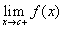(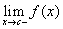).

It's easy enough to prove that all elementary functions are continuous whatever point you take in the domain. So in order to find interesting examples we'll often use other kinds of functions, mainly functions defined in pieces.

• Example 1: Consider the function f(x)=sgn(x). As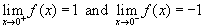, the limit as x approaches zero does not exist, so the function can't be continuous. If you examine the graph, you can easily understand what we mean when saying "the graph can't be drawn without lifting our pencil from the paper".
• Example 2: Consider the function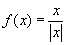and the point c=0. Does it make sense to investigate the continuity of this function at zero?. The answer is obviously not, because zero is not in the domain. Modify slightly the definition by considering the function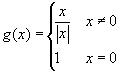. The question of continuity in zero now makes sense, but the function is not continuous, as, so that the limit does not exist. Going a step further we could consider a still slightly different situation: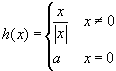. Now the question may be: can we find a value a for which the function is continuous? The answer is again negative, because the limit of the function can't exist, no matter what a is.
• Example 3: Consider the function: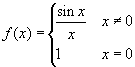. Now, as we know from a fundamental limit already discussed, the function is continuous in zero. Observe that if we change the value f(0) to something different the function is no more continuous: we have chosen f(0)=1 just to make the function continuous.
• Example 4: Consider the function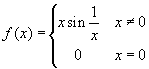. The function is continuous at zero: it is the product of x, that tends to zero, times a bounded function (see algebraic rules), so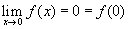. We may ask: can we draw the graph of this function without lifting our pencil from the paper? To answer this question we must before all calculate the length of the curve in the interval, say, [-1,1]: this length is infinite! So the rough definition given at the top of this page does not work in this case: the concept of continuity is not so obvious. See the graph in the picture here below.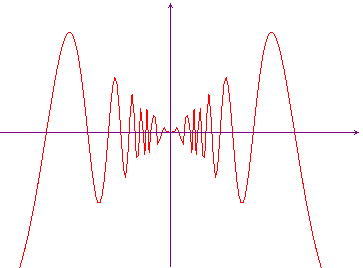• Example 5: Consider the function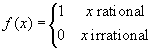. This function, often called Dirichlet's function, is continuous at no point in the real line: it's a very strange function! Its graph can only be imagined, and no computer display can visualize it, as the values of f jump unceasingly between 0 and 1.
• Example 6: Consider the function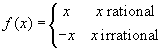. This is a modification of the previous one: it jumps unceasingly between the two bisectors y=x and y=-x. It is continuous only at zero (!) and obviously the problem of graphing it without lifting the pencil from the paper makes no sense, even if we consider only a small neighbourhood of zero.